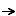Home

CUIPL3. Elemental PatternsDependency

Dependency

Problem

Items introduced by the user in the context of an event execution are not always independent of each other, specially when the items are conceptually related among them. For instance, some item values can be directly affected by values taken by others items. If the system is capable of dealing with these restrictions, the system will not allow the user to input combinations of invalid data.

Clip 1. Example of dependency.

Principle

Validation, error prevention

Forces

• Resolve & circular dependencies to avoid infinite loops.

Solution

The dependency pattern arises when the determination of a value (one or more arguments) leads to the variation of state (value or restrictions) in other arguments or items of data requested by the system. Then, we can say that the value of an item depends on another.

Rationale

Helps the user to understand the logic behind the software.

Impedes the introduction of well-know invalid combination of data.

Examples

Clip 1 shows an small example of dependency. The UI is designed to set the cost, margin and final price of a product. The user interface shows changes to any of these variables and their impact in the others.

There are two types of dependencies in the example:

1. Current values for cost, margin and price are recovered when the product is selected. This is a special case of State Recovery pattern where the object identity recovers object's properties.
2. Cost, margin, & price fields are related in terms of calculations. The dependencies are the following ones:
• Price = Cost * (1 + Margin)
• Margin = (Price - Cost) / Cost

Specification

Event-condition-action rules (for short ECA-rules) can be used to describe the dependencies and when to raise changes in the user interface. The semantic of an ECA-rule is the following: whenever an event is launched, the condition is evaluated, if it is satisfied, the corresponding action is executed.

The previous example shown in Clip 1 can be specified using three rules as follows:

Event: Cost.Changed
Condition: Cost.HasValue and Margin.HasValue
Action: Price.Value = Cost.Value * (1 + Margin.Value)

Event: Margin.Changed
Condition: Cost.HasValue and Margin.HasValue
Action: Price.Value = Cost.Value * (1 + Margin.Value)

Event: Price.Changed
Condition: Cost.HasValue
Action: Margin = (Price.Value - Cost.Value) / Cost.Value